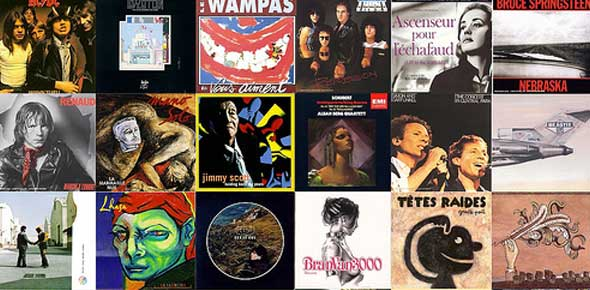# Properties Of H2O

5 Questions | Total Attempts: 112Settings3 States of Matter

Related Topics
• 1.
The three states of matter are...........?
• A.

Cold, hot, warm

• B.

Solid, liquid, gas

• C.

• D.

None of the above

• 2.
At what temperature does water turn to ice?
• A.

0 degrees Celcius

• B.

2 degrees Celcius

• C.

100 degrees Celcius

• D.

None of the above

• 3.
What is the boiling point of water?
• A.

0 degrees Celcius

• B.

2 degress Celcius

• C.

100 degrees Celcius

• D.

None of the above

• 4.
Which of the following is a gas?
• A.

Hydrogen

• B.

Oxygen

• C.

Nitrogen

• D.

All of the above

• 5.
What are the properties of gases?
• A.

Definite shape & volume

• B.

No definite shape or volume

• C.

Both A & B

• D.

Niether A or B